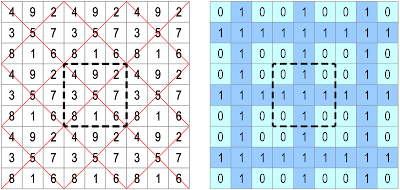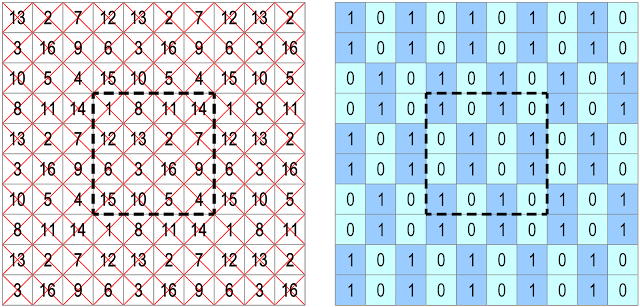## Monday, 2 September 2019

### Even and Odd Number Patterns on Magic Tori of Orders 3 and 4

Ancient references to the pattern of even and odd numbers of the 3 × 3 magic square appear in the "I Ching" or "Yih King" (Book of Changes). In the introduction to the Chou edition, the scroll of the river Loh or "Loh-Shu", which is represented by the magic square of order-3, is written with black dots (the yin symbol and emblem of earth) for the even numbers, and white dots (the yang symbol and emblem of heaven) for the odd numbers. The "Loh-Shu" is incorporated in the writings of Ts'ai Yuän-Ting who lived from 1135 to 1198:The Scroll of Loh, according to Ts'ai Yüang-Ting

A normal magic torus (or magic square) of order-n uses consecutive numbers from 1 to n² and consequently, for the order-3, the magic constant (MC) = 15. The Loh-Shu Magic Torus of Order-3 is presented below, together with its corresponding even and odd number pattern P3:The "Loh-Shu" Magic Torus of Order-3 and its Even and Odd Number (x mod 2) Pattern P3

The magic torus T3 of order-3 is shown here as seen from the Scroll of Loh magic square viewpoint.

The even and odd number pattern P3 has reflection symmetry (with 6 lines of symmetry); rotational symmetry (of order 4); and point symmetry (over number 5, between numbers 3 and 7, between numbers 1 and 9, and between numbers 4, 6, 2, and 8).

## The Even and Odd Number Patterns of the Magic Tori of Order-4

Only consecutively numbered magic tori (with numbers from 1 to n²) are studied here, and therefore, for order-4, the magic constant MC = 34.

The "Table of Fourth-Order Magic Tori" lists and describes each magic torus of order-4 using index numbers, while "255 Fourth-Order Magic Tori, and 1 Third-Order Magic Torus" gives a group categorisation of the magic tori of order-4 using type numbers. Both of these classifications give the details of the Frénicle indexed magic squares that are displayed by each torus.

In the study that follows it can be seen that there are 4 essentially different even and odd number patterns on the magic tori of order-4:

### The Order-4 Even and Odd Number Pattern P4.1 (represented by the pandiagonal torus T4.198)The Pandiagonal Torus T4.198 of Order-4 and its Even and Odd Number (x mod 2) Pattern P4.1

The pandiagonal torus of order-4 (with index n° T4.198 and type n° T4.01.2) is shown here as seen from the Frénicle n° 107 magic square viewpoint.

The even and odd number pattern P4.1 has reflection symmetry (with 6 lines of symmetry); rotational symmetry (of order 2); and multiple point symmetry when reading as a torus. The pattern P4.1 also has negative symmetry (evens to odds and vice versa).

The even and odd number pattern P4.1 concerns 79 out of the 255 magic tori, and 404 out of the 880 magic squares of order-4. These include the 3 pandiagonal tori that display 48 pandiagonal squares; 30 semi-pandiagonal tori that display 240 semi-pandiagonal squares; 12 partially pandiagonal tori that display 48 partially pandiagonal squares; and also 34 basic magic tori that display 68 basic magic squares.

### The Order-4 Even and Odd Number Pattern P4.2 (represented by the semi-pandiagonal torus T4.059)The Semi-Pandiagonal Torus T4.059 of Order-4 and its Even and Odd Number (x mod 2) Pattern P4.2

The semi-pandiagonal torus of order-4 (with index n° T4.059 and type n° T4.02.2.01) is shown here as seen from the Frénicle n° 21 magic square viewpoint.

The even and odd number pattern P4.2 has reflection symmetry (with 8 lines of symmetry); rotational symmetry (of order 4); and multiple point symmetry when reading as a torus. The pattern P4.2 also has negative symmetry (evens to odds and vice versa).

The even and odd number pattern P4.2 concerns 72 out of the 255 magic tori, and 256 out of the 880 magic squares of order-4. These include 18 semi-pandiagonal tori that display 144 semi-pandiagonal squares; 6 partially pandiagonal tori that display 16 partially pandiagonal squares; and also 48 basic magic tori that display 96 basic magic squares.

### The Order-4 Even and Odd Number Pattern P4.3 (represented by the partially pandiagonal torus T4.186)The Partially Pandiagonal Torus T4.186 of Order-4 and its Even and Odd Number (x mod 2) Pattern P4.3

The partially pandiagonal torus (with index n° T4.186 and type n° T4.03.1.3) is shown here as seen from the Frénicle n° 100 magic square viewpoint.

The even and odd number pattern P4.3 has reflection symmetry (with 8 lines of symmetry); rotational symmetry (of order 4); and multiple point symmetry when reading as a torus. The pattern P4.3 also has negative symmetry (evens to odds and vice versa).

The even and odd number pattern P4.3 concerns 16 out of the 255 magic tori, and 44 out of the 880 magic squares of order-4. These include 6 partially pandiagonal tori that display 24 partially pandiagonal squares; and also 10 basic magic tori that display 20 basic magic squares.

### The Order-4 Even and Odd Number Pattern P4.4 (represented by the basic magic torus T4.062)The Basic Magic Torus T4.062 of Order-4 and its Even and Odd Number (x mod 2) Pattern P4.4

The basic magic torus (with index n° T4.062 and type n° T4.05.1.12) is shown here as seen from the Frénicle n° 37 magic square viewpoint.

The even and odd number pattern P4.4 has reflection symmetry (with 8 lines of symmetry); rotational symmetry (of order 2); and multiple point symmetry when reading as a torus. The pattern P4.4 also has negative symmetry (evens to odds and vice versa).

The even and odd number pattern P4.4 concerns 88 out of the 255 magic tori, and 176 out of the 880 magic squares of order-4. All of these 88 magic tori and the displayed 176 magic squares are basic magic.

### Observations on the Even and Odd Number Patterns of the Magic Tori and Magic Squares of Order-4

The findings of the even and odd number patterns of the magic tori of order-4 are recapitulated and analysed in comparative tables, (together with the Dudeney complementary number patterns and the Magic Torus self-complementary and paired complementary number patterns), in the file below:

## The Even and Odd Number Patterns of the Semi-Magic Tori of Order-4

Only consecutively numbered semi-magic tori (with numbers from 1 to n²) are studied here, and consequently, for the order-4, the magic constant MC = 34.

In "255 Fourth-Order Magic Tori, and 1 Third-Order Magic Torus" the 4,038 semi-magic tori of order-4 have been sorted, and type numbers have been attributed taking into account the arrangements of their magic diagonals (if any).

Each of the four even and odd number patterns already found above on the magic tori of order-4, also occur on semi-magic tori of order-4. For example, from left to right below, we find a semi-magic torus type T4.08.0W with pattern P4.1; a semi-magic torus type T4.08.0X with pattern P4.2; a semi-magic torus type T4.07.0Y with pattern P4.3; and a semi-magic torus type T4.09.00Z with pattern P4.4:Semi-Magic Tori of Order-4 with Even and Odd Number (x mod 2) Patterns P4.1, P4.2, P4.3 and P4.4

The even and odd number patterns P4.1 to P4.4 are not the only ones that can be found, but the patterns which are specific to the semi-magic tori of order-4 have neither been fully investigated nor precisely counted, and the examples that follow only represent some of the different varieties.

### The Order-4 Even and Odd Number Pattern P4.5 (represented by the semi-magic torus type T4.06.0A)The Semi-Magic Torus type T4.06.0A of Order-4 and its Even and Odd Number (x mod 2) Pattern P4.5

This semi-magic torus type n° T4.06.0A was found by Walter Trump, and has been previously illustrated in "255 Fourth-Order Magic Tori, and 1 Third-Order Magic Torus".

The even and odd number pattern P4.5 has diagonal reflection symmetry (with 4 lines of symmetry); and negative (evens to odds and vice versa) rotational symmetry (of order 2). The blocks of even and odd numbers are translations of each other.

### The Order-4 Even and Odd Number Pattern P4.6 (represented by the semi-magic torus type T4.07.0A)The Semi-Magic Torus type T4.07.0A of Order-4 and its Even and Odd Number (x mod 2) Pattern P4.6

This semi-magic torus type n° T4.07.0A was found by Walter Trump, and has been previously illustrated in "255 Fourth-Order Magic Tori, and 1 Third-Order Magic Torus".

The even and odd number pattern P4.6 has reflection symmetry (with 4 lines of symmetry); rotational symmetry (of order 2); and multiple point symmetry when reading as a torus. The blocks of even and odd numbers are translations of each other.

### The Order-4 Even and Odd Number Pattern P4.7 (represented by the semi-magic torus type T4.09.00M)The Semi-Magic Torus type T4.09.00M of Order-4 and its Even and Odd Number (x mod 2) Pattern P4.7

This semi-magic torus type n° T4.09.00M was found by Walter Trump, and has been previously illustrated in "255 Fourth-Order Magic Tori, and 1 Third-Order Magic Torus".

The even and odd number pattern P4.7 has both positive and negative reflection symmetry; negative rotational symmetry (of order 2); and multiple negative point symmetry when reading as a torus. The blocks of even and odd numbers are translations of each other.

### The Order-4 Even and Odd Number Pattern P4.8 (represented by the semi-magic torus type T4.10.000X)The Semi-Magic Torus type T4.10.000X of Order-4 and its Even and Odd Number (x mod 2) Pattern P4.8

3,726 semi-magic tori of the type 10 have been found by Walter Trump, and his findings are detailed in "255 Fourth-Order Magic Tori, and 1 Third-Order Magic Torus".

The T4.10.000X's even and odd number pattern P4.8 has negative reflection symmetry (2 lines of symmetry); negative rotational symmetry (of order 2); and multiple point symmetry when reading as a torus. The blocks of even and odd numbers are translations of each other.

## General Observations

The "Table of Fourth-Order Magic Tori" has been revised to include the even and odd number pattern references for each of the magic tori of order-4 (listed together with the Frénicle indexed magic squares that each magic torus displays).

Other interesting websites that examine, but do not enumerate, the different even and odd number patterns on magic squares of order-4, include "4x4 Magic Squares" by Dan Rhett and "Pattern in Magic Squares" by Vipul Chaskar.

A brief look at higher-orders suggests that the order-5 has many irregular patterns; the order-6 has many patterns with negative reflection symmetry (evens to odds and vice versa); the order-7 has many patterns with rotational symmetry; and the order-8 has patterns very like those of the order-4 examined above. All of these orders merit an in-depth analysis of their even and odd number patterns.

In conclusion, it is interesting to note that the Arab mathematicians of the tenth century constructed odd-order magic squares with striking even and odd number patterns (as for example the magic squares in figures b44 and b49 on pages 243 and 248 of Magic Squares in the Tenth Century: Two Arabic treatises by Anṭākī and Būzjānī, translated by Jacques Sesiano). And in the same context, Paul Michelet has recently brought to my attention a magnificent 15 x 15 magic square by Ali b. Ahmad al-Anṭākī (d. 987). Al-Anṭākī's bordered magic square is solid evidence of the Middle Eastern mathematicians' mastery of even and odd number patterns!